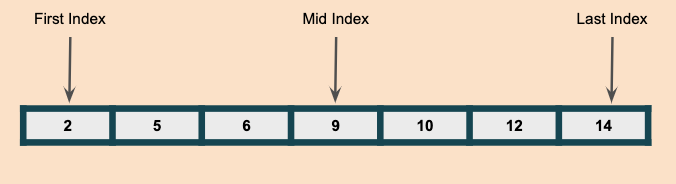# Binary Search Algorithm In JavaBinary Search is a Logarithmic search which finds the target element in a sorted array in O(logN) times compared to linear search which takes O(N).

Binary search uses divide and conquer approach and can only work on a sorted array or list.

In this approach, we divide the input collections into two equal halves using the first index and last index, for every iteration we will divide into much smaller equal partitions.

Algorithm:

Step1: we calculate mid index using the first and last index [(firstIndex + lastIndex)/2].

Step2: we compare the target value with mid-index value if mid is equal target value we return mid-index, else if mid is less than target value we update the first index to mid +1 index and if mid is greater than the last index we will update the last index to mid -1.

Step3: Repeat Step1 & Step2 until we find the target or if first index is greater than last index value.

With this approach we are eliminating the elements to search by half every time we didn’t find the target, so the worst case would be logN times.

Example:
Let’s take the below array of sorted numbers and the target to search is 14, in a regular linear approach it will take O(N) times to find the target element.

With a binary search approach, we will start with the mid element that itself reduces the number of elements to search by half.

Iteration 1:

In the first iteration, we compare the mid element at index 3 which is 9 with target element 14, as said in Step 2, since mid-index value is less than the target we will change the first index/left index to mid +1 which is 4.

Iteration 2:

In the second iteration, the mid index would be 5 and we compare the mid index value which is 12 with 14, we repeat Step 2 since it is less than target 14 we change the first index/ left index to mid +1 which is 6.

Iteration 3:

In the third iteration, the mid index is 6 where the value at mid index is equal to target and we found the target and we return.

The max comparisons we did is 3 which is less than O(logN)

Solution:

``````class HackerHeap {
public int search(int[] nums, int target) {
int left = 0;
int right = nums.length -1;
while(left<=right){
int mid = (left+right)/2;
if(nums[mid] == target) return mid;
else if(nums[mid]>target) right = mid-1;
else left = mid+1;
}

return -1;
}
}``````

Here is the video explanation of binary search